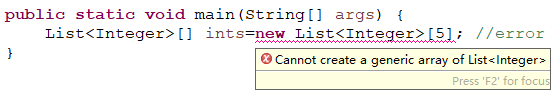# 尝试创建一个泛型数组# 泛型与类型擦除

Java引入泛型的目的之一是指定容器要装载的对象的类型，由编译器来保证类型的正确性。这样能一定程度保证代码的安全性。

	public static void main(String[] args) {
List<Integer> list=new ArrayList<>();
}


	public static void main(String[] args) {
Class ca=new HashSet<Integer>().getClass();
Class cb=new HashSet<String>().getClass();
System.out.println(ca==cb);  //true
}


Java的泛型的实现依赖于 类型擦除 ，所谓擦除，就是在使用泛型时，类型参数(正如上面的Integer和String)会被自动忽略，因此，在真正运行泛型代码的时候，ca和cb都是原生的HashSet类型，或者你可以认为它们都是HashSet< Object>类型(这种只包含类型< T>的泛型会被擦除为最大的父类Object)

	public static void main(String[] args) {
Class ca=new HashSet<Integer>().getClass();
Class cb=new HashSet<String>().getClass();
System.out.println(ca);  //class java.util.HashSet
}


# 数组与泛型

	public static void main(String[] args) {
List<Integer>[] ints;  //泛型数组引用
List[] ls=new List; //非泛型数组
ints=ls;               //赋值，会自动转型
for(List l:ints) {
l=new ArrayList<>();
}
}


	public static void main(String[] args) {
T[] t=new T; //T cannot be resolved to a type
//error
}


	public static void main(String[] args) {
Integer[] intA= {1,2,3,4};
ArraryT<Integer> arrayT=new ArraryT<>();
Integer[] ints=arrayT.getArrayT(intA);
for(Integer i:ints) {
System.out.print(i);  //1234
}
}

//ArrayT类
public class ArraryT<T> {
public T[] getArrayT(T[] t) {
return t;
}
}


06-155907
07-27243706-154618
08-081214
05-05102
11-271692
08-17